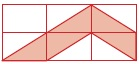# User Forum

Subject :NCO    Class : Class 3

If I shade 2 more small rectangles in the given figure, what fraction of the figure will be unshaded?(a) 1/4
(b) 1/2
(c) 3/4
(d) 5/4

explain this question

Class : Class 3
a

## Ans 2:

Class : Class 6
Look 6 small rectangles are there. The shaded part is - (half+half)+(half+half)+half small rectangles =(1+1)+half small rectangles=2+half small rectangles=2 and half small rectangles.You want to shade 2 more small rectangles.Then the shaded part will be=2 and half +2 small rectangles= 4 and half small rectangles. Then the unshaded part will be =6 - 4 and half small rectangles=1 and half small rectangles.The fraction of unshaded small rectangles will be =1 and half by 6=3 by 12=2 by 8=1 by 4. So the answer will be (a) 1 by 4

Class : Class 3
a

Class : Class 4
b

Class : Class 4

## Ans 6:

Class : Class 3
Look 6 small rectangles are there. The shaded part is - (half+half)+(half+half)+half small rectangles =(1+1)+half small rectangles=2+half small rectangles=2 and half small rectangles.You want to shade 2 more small rectangles.Then the shaded part will be=2 and half +2 small rectangles= 4 and half small rectangles. Then the unshaded part will be =6 - 4 and half small rectangles=1 and half small rectangles.The fraction of unshaded small rectangles will be =1 and half by 6=3 by 12=2 by 8=1 by 4. So the answer will be (a) 1 by 4

## Ans 7:

Class : Class 6
Sorry DHRUV.JAIN, My answer is wrong because there are 6 small rectangles. 5 parts are shaded in the in the big rectangle. The answer is 5/6. The option is (D)

Class : Class 4
c